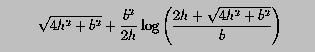# Programming Example: The Length of a Parabola Segment### Problem Statement

Given base b and height h, the length of a special segment on a parabola can be computed as follows:Write a program to read in the values of base and height, and use the above formula to compute the length of the parabola segment. Note that both base and height values must be positive.### Solution

```! -----------------------------------------------------------
!   Calculate the length of a parabola given height and base.                                         *
! -----------------------------------------------------------

PROGRAM  ParabolaLength
IMPLICIT  NONE

REAL  :: Height, Base, Length
REAL  :: temp, t

WRITE(*,*)  'Height of a parabola : '

WRITE(*,*)  'Base of a parabola   : '

! ... temp and t are two temporary variables

t      = 2.0 * Height
temp   = SQRT(t**2 + Base**2)
Length = temp + Base**2/t*LOG((t + temp)/Base)

WRITE(*,*)
WRITE(*,*)  'Height = ', Height
WRITE(*,*)  'Base   = ', Base
WRITE(*,*)  'Length = ', Length

END PROGRAM  ParabolaLength
```
Click here to download this program.### Program Output

``` Height of a parabola :
100.0

Base of a parabola   :
78.5

Height = 100.
Base   = 78.5
Length = 266.149445
```

The input values for Height and Base are 100.0 and 78.5, respectively. The computed length is 266.149445.### Discussion

• The values of base and height will be stored in REAL variables Base and Height, respectively. Length will be used to store the parabola segment length.
• Since the content in the square root is used twice, it would be more convenient to save the result in a variable. This value will be stored in temp. Since 2h also appears a few times, variable t is used to store this value. After reading in Height and Base, 2.0 * Height is computed and stored in t with the first assignment. Then, the second assignment computes the content in the square root and stores the result into temp.
• The third assignment compute the segment length and stores the result into Length. Note that intrinsic function LOG() is used.
• The four WRITE statements display the input and the results.